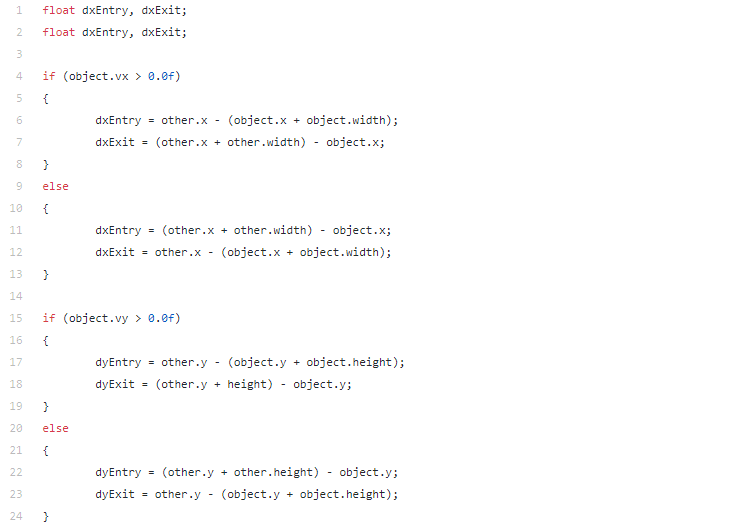# Using Swept AABB to detect and process collision

Updated: Jul 2, 2021Level: Very Beginner

### What is AABB?

AABB stands for Axis-Aligned Bounding Box, it is an algorithm to detect collision between a rectangle’s edges, in this case, those edges are parallel with coordinate axes. Basically, we will check two rectangles overlap with each other or not.

### What is Swept AABB?

We will have some problems if we use AABB, let’s see this example:In the first frame, rectangle 1 doesn’t collide with a blue rectangle. And next frame, the rectangle 1 move to the next position, but velocity too fast and make it go through the blue rectangle. If we check collision at this frame, rectangle 1 doesn’t collide with the blue one.

Let’s think if we can predict the next position in the next frame, we will know and prevent our object from this problem. This is called Swept AABB.

### AABB Collision

To check 2 rectangles are overlapped each other or not, we will check each pair of corresponding edges.We always have this at the same time:

• other.left <= object.right

• other.right >= object.left

• other.top >= object.bottom

• other.bottom <= object.top

If one of these conditions is wrong, so 2 rectangles aren’t overlapped.In this example, we have ‘left’ of ‘other’ greater than ‘right’ of ‘object’.

This is a simple function to check collision:We can invert conditions to check faster.

### Using Swept

First, we calculate the distance between the corresponding edges.In this,

• dxEntry, dyEntry: the distance need to move to begin contact

• dxExit, dyExit: the distance need to move to exit contact

We also need to check the sign of velocity and calculate the distance in each caseNext, from distance and velocity, we can calculate the time

### || time = distance / velocityIn order to collide, both axes x and y need to get collided. So, we will take the longest time to begin collision.

And when end collision, we only need one of the axes exit collision, so we will take the quickest time to exit collision.We can check collision by:

• time to begin collision from 0 to 1

• time to end collision must be bigger than time to begin collision.

Finally, we will return time to begin a collision.

### Handle collision

We can use it to calculate the distance need to move to the next frame based on the returned time.To optimize, we can do one more step by using a rectangle made from the position at the current frame and the next frame to check collision with another object.We put this code at the top of Swept AABB function.### Detect collision directionThat’s it! This algorithm is very simple, right?

I hope this basic concept can help you in your project and move to the more difficult algorithms like SAT (Separating Axis Theorem).

See All